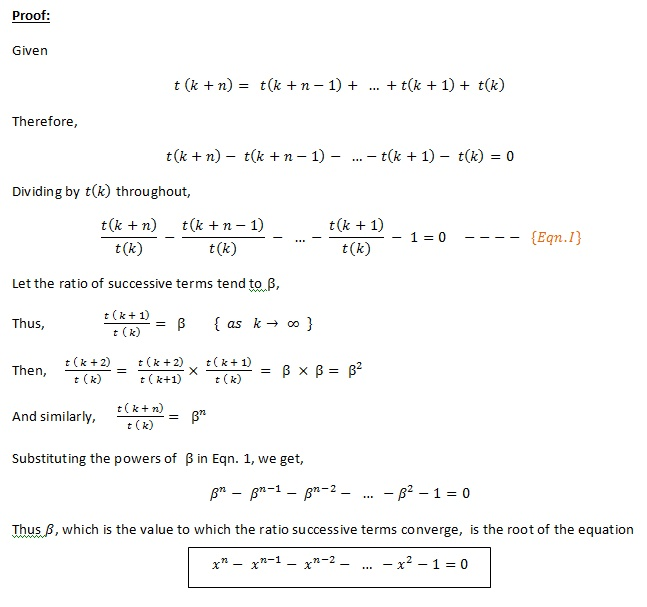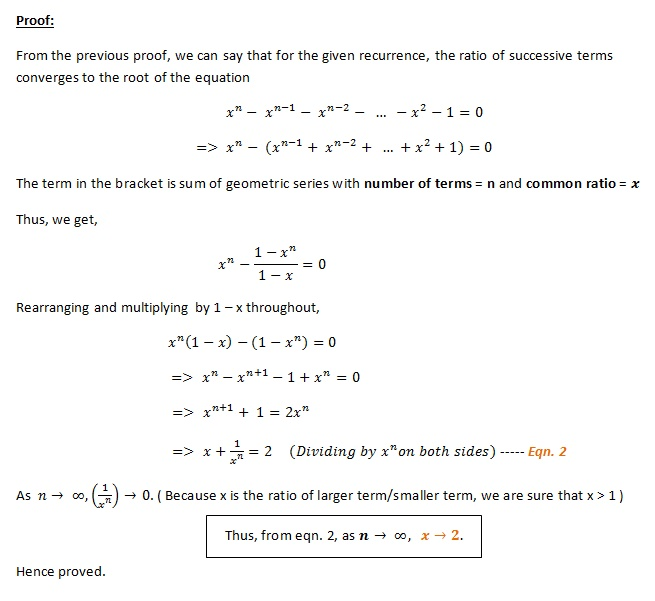# Fibonacci-like sequences and ratio of successive terms

Page 1  |  Page 2   |  Page 3 of 3

First, we prove that ratio of successive terms for a recurrence of order n approaches the root of the equation x^n - x^(n-1) - x^(n-2) - ... - 1 = 0.
Claim: Given a recurrence of order n, such that n-th term t(k + n) = t(k + n - 1) + .... + t(k + 1) + t(k), then the ratio of successive terms converges to the root of the equation x^n - x^(n-1) - x^(n-2) - ... - 1 = 0.

Proof:
See image below. Proof is trivial.Next, we prove that ratios themselves approach 2 as the order of the recurrence (n) tends to infinity.
Claim:Given a recurrence of order n, such that n-th term t(k+n) = t(k+n-1) + .... + t(k+1) + t(k), then the ratio of successive terms approaches 2 as n and k, both tend to infinity.

Proof: See image below.Page 1  |  Page 2   |  Page 3 of 3
 Quick Comment : * Name Email ( Your email id is safe with us! ) * Comments * Type the number of characters in the word "HUMAN"